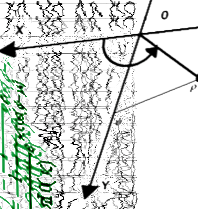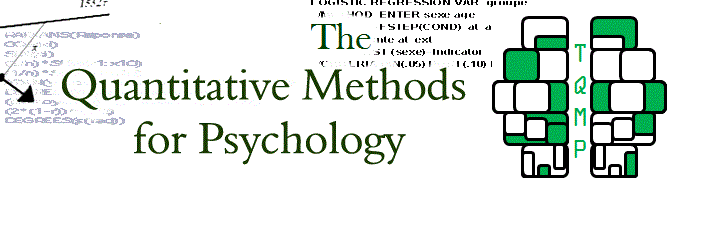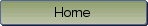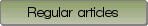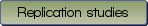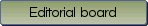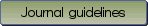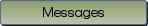Standard errors: A review and evaluation of standard error estimators using Monte Carlo simulations

Bibliographic information: BibTEX format RIS format XML format APA style
Cited references information: BibTEX format APA style

Harding, Bradley , Tremblay, Christophe , Cousineau, Denis
107-123
Keywords: Statistics , Standard errors , Estimation , Evaluation
(no sample data)   (no appendix)

Characteristics of a population are often unknown. To estimate such characteristics, random sampling must be used. Sampling is the process by which a subgroup of a population is examined in order to infer the values of the population's true characteristics. Estimates based on samples are approximations of the population's true value; therefore, it is often useful to know the reliability of such estimates. Standard errors are measures of reliability of a given sample's descriptive statistics with respect to the population's true values. This article reviews some widely used descriptive statistics as well as their standard error estimators and their confidence intervals. The statistics discussed are: the arithmetic mean, the median, the geometric mean, the harmonic mean, the variance, the standard deviation, the median absolute deviation, the quantile, the interquartile range, the skewness, as well as the kurtosis. Evaluations using Monte-Carlo simulations show that standard errors estimators, assuming a normally distributed population, are almost always reliable. In addition, as expected, smaller sample sizes lead to less reliable results. The only exception is the estimate of the confidence interval for kurtosis, which shows evidence of unreliability. We therefore propose an alternative measure of confidence interval based on the lognormal distribution. This review provides easy to find information about many descriptive statistics which can be used, for example, to plot error bars or confidence intervals.

Be informed of the upcoming issues with RSS feed:RSS